Next: 4.8.4 Preservation of Voids Up: 4.8 Void Detection Previous: 4.8.2 Graph Setup Algorithm

## 4.8.3 Algorithmic Complexity

The setup of the graph requires a sequential traversal over the H-RLE data structure. A first order finite difference stencil is used to enable fast access to neighbor grid points. Hence, the neighbor grid points of the first point of each segment can be tested for connectivity in constant time. As a consequence, the overall complexity required to set up the reduced graph exhibits a linear scaling with the number of defined grid points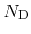. For the size of the reduced graph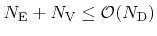holds, since each segment in the H-RLE data structure leads to the insertion of, at most, one vertex and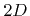edges, if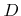is the number of dimensions. As previously mentioned, the connected components of a graph can be obtained with linear complexity, which leads to an overall algorithmic complexity of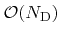.

The memory requirements are also optimal. For each segment of the H-RLE data structure a reference of the corresponding vertex must be stored. The memory requirements for the reduced graph can usually be neglected, because, in practice, the number of vertices is much smaller than the number of segments.Next: 4.8.4 Preservation of Voids Up: 4.8 Void Detection Previous: 4.8.2 Graph Setup Algorithm

Otmar Ertl: Numerical Methods for Topography Simulation